Courses

# Engineering Mechanics Civil Engineering (CE) Notes | EduRev

## Civil Engineering (CE) : Engineering Mechanics Civil Engineering (CE) Notes | EduRev

The document Engineering Mechanics Civil Engineering (CE) Notes | EduRev is a part of the Civil Engineering (CE) Course Topic wise GATE Past Year Papers for Civil Engineering.
All you need of Civil Engineering (CE) at this link: Civil Engineering (CE)

Question 1: A cable PQ of length 25 m is supported at two ends at the same level as shown in the figure. The horizontal distance between the supports is 20 m. A point load of 150 kN is applied at point R which divides it into two equal parts.    [2018 : 2 Marks, Sel-II]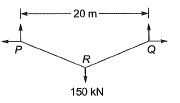Neglecting the self-weight of the cable, the tension (in kN, integer value) in the cable due to the applied load will be ________.

Solution:
Method-I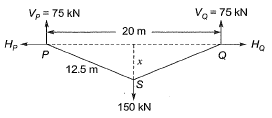12.52 = x2 + 102
⇒ x = 7.5 m
Bending moment at S = 0 (Consider the left part)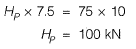Tension in cable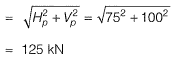Method-ll
Using sine rule at joint S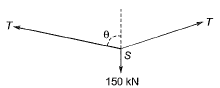Calculating θ,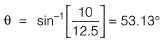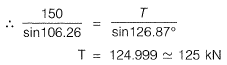Question 2: Two rigid bodies of mass 5 kg and 4 kg are at rest on a frictionless surface until acted upon by a force of 36 N as shown in the Figure. The contact force generated between the two bodies is    [2018 : 2 Marks, Set-II]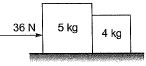(a) 4.0 N
(b) 7.2 N
(c) 9.0 N
(d) 16.0 N
Solution: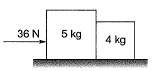As after action of 36 N, both blocks will move with same acceleration so considering 5 kg and 4 kg together in a system and applying Newton’s 2nd law.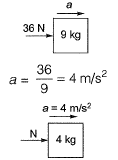N = ma {Newton’s 2nd law}
N= 4 x 4 = 16 N

Question 3: A cylinder of radius 250 mm and weight, W= 10 kN is rolled up an obstacle of height 50 mm by applying a horizontal force P at its centre as shown in the figure.    [2018 : 2 Marks, Set-I]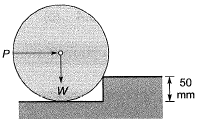All interfaces are assumed frictionless. The minimum value of P is
(a) 4.5 kN
(b) 5.0 kN
(c) 6.0 kN
(d) 7.5 kN
Solution: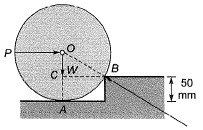Given: r = 250 mm, W = 10 kN
Note:
1. When the cylinder will be about to move out of the cylinder, it will loose its contact at point A, only contact will be at point B.
2. Considering equation of cylinder of that instant under P, W and RB (contact force at B).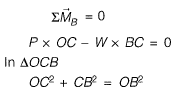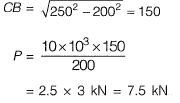Question 4: An aircraft approaches the threshold of a runway strip at a speed of 200 km/h. The pilot decelerates the aircraft at a rate of 1.697 m/s2 and takes 18 s to exit the runway strip. If the deceleration after exiting the runway is 1 m/s2, then the distance (in m, up to one decimal place) of the gate position from the location of exit on the runway is ____    [2018 : 2 Marks, Set-I]
Solution: Speed of aircraft,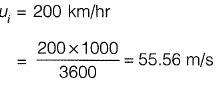Deceleration of aircraft on the runway
= 1.697 m/s2
Aircraft takes 18 sec to exit the runway strip. Speed of the aircraft at the exit of the runway
uf = ui + at
= 55.56 - 1.697 x 18
= 25.014 m/s
After runway aircraft decelerate with 1 m/s2
Total distance travelled by the aircraft from the location of exit on the runway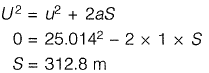Question 5: A particle of mass 2 kg is travelling at a velocity of 1.5 m/s. A force f (t) = 3t2 (in N) is applied to it in the direction of motion for a duration of 2 seconds, where f denotes time in seconds. The velocity (in m/s, up to one decimal place) of the particle immediately after the removal of the force is ___________.    [2017 : 2 Marks, Set-I]
Solution: m = 2 kg, V0 = 1.5 m/sec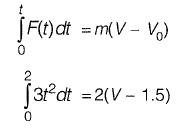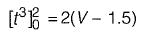⇒ (8 - 0) = 2 (V - 1.5)
V = 5.5 m/s

Question 6: The reaction rate involving reactants A and B is given by -k [A]α [B]β.  Which one of the following statements is valid for the reaction to be a first- order reaction?    [2017 : 1 Mark, Set-I]
(a) α = 0 and β = 0
(b) α = 1 and β = 0
(c) α = 1 and β = 1
(d) α = 1 and β = 2

Solution: The order of reaction is the exponent to which its concentration term in the rate equation is raised.
Rate, r = k [A]α [B]β
For first order reaction,
α + β = 1

Question 7: The magnitudes of vectors P, Q and R are 100 kN, 250 kN and 150 kN, respectively as shown in the figure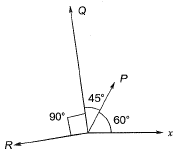The respective values of the magnitude (in kN) and the direction (with respect to the x-axis) of the resultant vector are     [2016 : 2 Marks, Set-I]
(a) 290,9 and 96.0°
(b) 368.1 and 94.7°
(c) 330.4 and 118.9°
(d) 400.1 and 113.5°
Solution: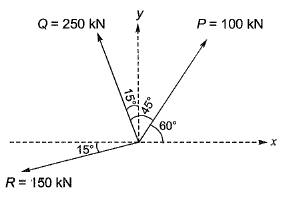Resultant force in x-direction,
Rx = P cos60° - Q sin15° - R sin15°
= 100 cos60° - 250 sin15° - 150 cos15°
= -159.59 kN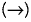= 159.59 kN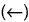Resultant force in y-direction,
Ry = Psin60° + Qcos15° - Rsin15°
= 100 sin60° + 250 cos15° - 150 sin15°
= 289.26 kN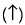Final resultant force,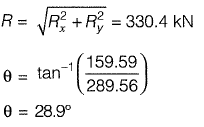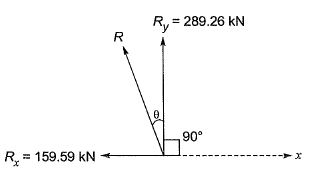Direction with respect to x-axis
= θ + 90°
= 28.9° + 90°
= 118.9°

Question 8: An assembly made of a rigid arm A-B-C hinged at end A and supported by an elastic rope C-D at end C is shown in the figure. The members may be assumed to be weightless and the lengths of the respective members are as shown in the figure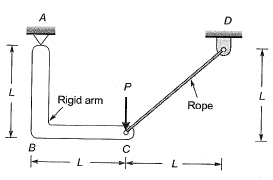Under the action of a concentrated load P at Cas shown, the magnitude of tension developed in the rope is    [2016 : 1 Mark, S e t-II]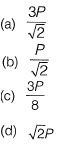Solution:
Method-I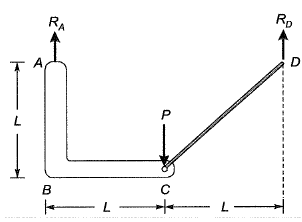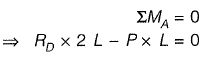⇒ RD = P/2
At joint D: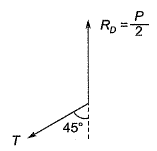∑Fy = 0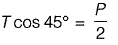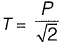Method-ll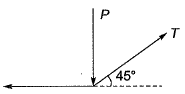Using sine rule at joint C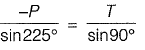∴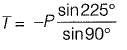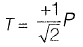Question 9: Polar moment of inertia (Ip), in cm4, of a rectangular section having width, b = 2 cm and depth, d = 6 cm is _____________.    [2014 : 1 Mark, Set-II]
Solution:
Polar moment of inertia is measure of an object’s ability to resist torsion under specified axis when and torque is being applied. It is also known as “second moment of area, “area moment of inertia”, “polar moment of area” or “second area moment”. Polar moment of inertia,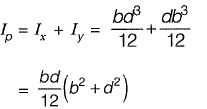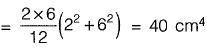Question 10: A disc of radius r has a hole of radius r/2 cut-out as shown. The centroid of the remaining disc (shaded portion) at a radial distance from the centre “O” is     [2010:2 Marks]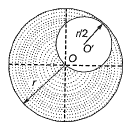(a) r/2
(b) r/3
(c) r/6
(d) r/8
Solution: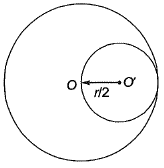The centroid of the shaded portion of the disc is given by,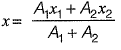where x is the radial distance from O.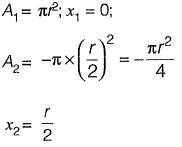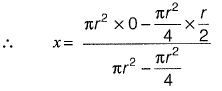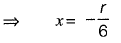Offer running on EduRev: Apply code STAYHOME200 to get INR 200 off on our premium plan EduRev Infinity!

,

,

,

,

,

,

,

,

,

,

,

,

,

,

,

,

,

,

,

,

,

;